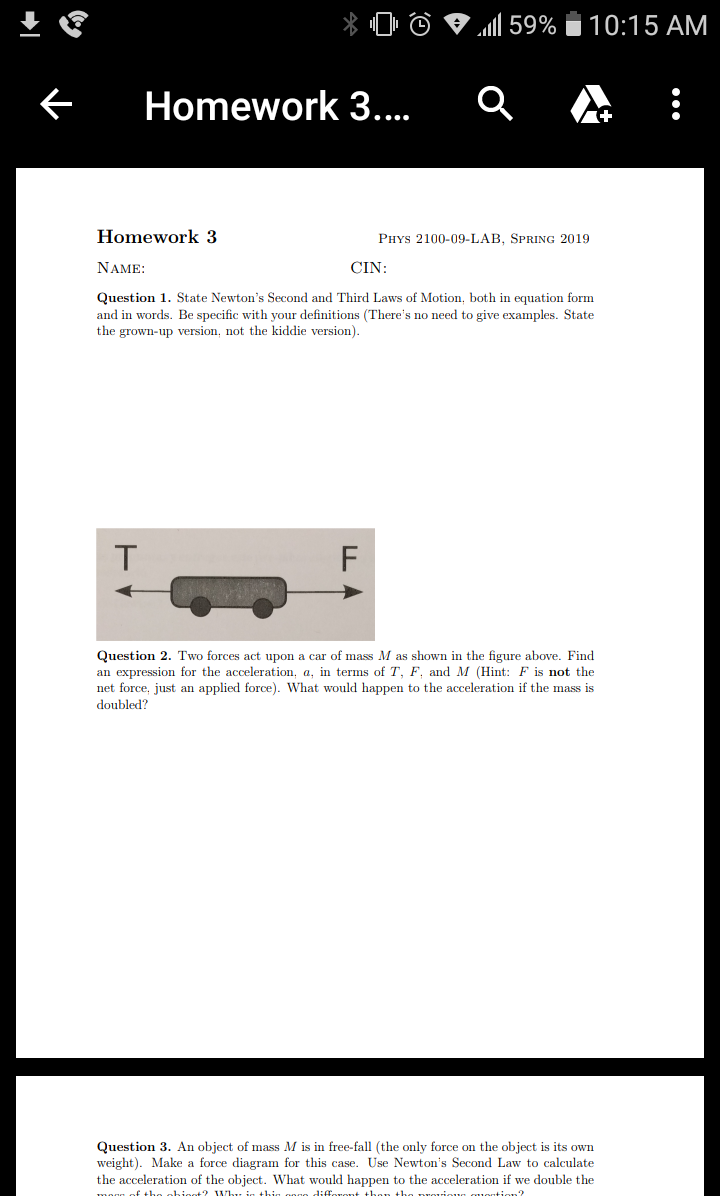# Homework 3Homework 3PHYs 2100-09-LAB, SPRING 2019NAMECINQuestion 1. State Newton's Second and Third Laws of Motion, both in equation formand in words. Be specific with your definitions (There's no need to give examples. Statethe grown-up version, not the kiddie version)Question 2. Two forces act upon a car of mass M as shown in the figure above. Findan expression for the acceleration, a, in terms of T, F. and M (Hint: F is not thenet force, just an applied force). What would happen to the acceleration if the mass isdoubled?Question 3. An object of mass M is in free-fall (the only force on the object is its ownweight). Make a force diagram for this case. Use Newton's Second Law to calculatethe acceleration of the object. What would happen to the acceleration if we double the

Question
12 views

Question 2help_outlineImage TranscriptioncloseHomework 3 Homework 3 PHYs 2100-09-LAB, SPRING 2019 NAME CIN Question 1. State Newton's Second and Third Laws of Motion, both in equation form and in words. Be specific with your definitions (There's no need to give examples. State the grown-up version, not the kiddie version) Question 2. Two forces act upon a car of mass M as shown in the figure above. Find an expression for the acceleration, a, in terms of T, F. and M (Hint: F is not the net force, just an applied force). What would happen to the acceleration if the mass is doubled? Question 3. An object of mass M is in free-fall (the only force on the object is its own weight). Make a force diagram for this case. Use Newton's Second Law to calculate the acceleration of the object. What would happen to the acceleration if we double the fullscreen
check_circle

Step 1

Equation for net force acting on the car from Newton’s second law is,

Step 2

a  is the acceleration of car.

There are two forces, T and F. These forces are in opposite direction. The arrow representing T is smaller than F. It means that F has greater magnitude than T.

Equation for Fnet is,

Step 3

Substitute the above equation ...

### Want to see the full answer?

See Solution

#### Want to see this answer and more?

Solutions are written by subject experts who are available 24/7. Questions are typically answered within 1 hour.*

See Solution
*Response times may vary by subject and question.
Tagged in

### Newtons Laws of Motion